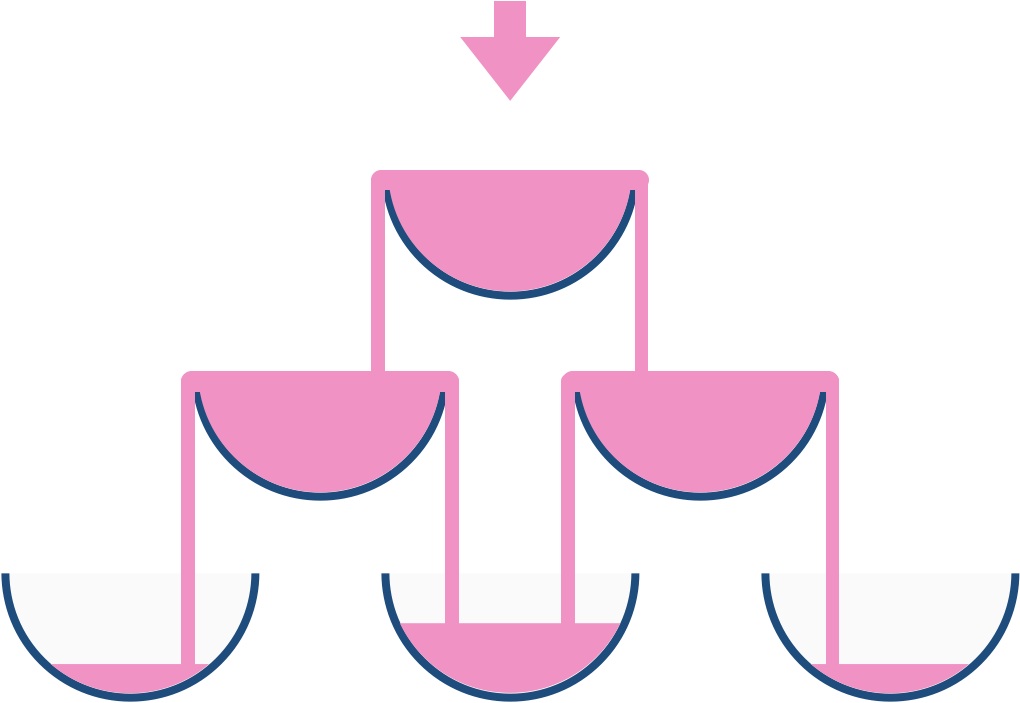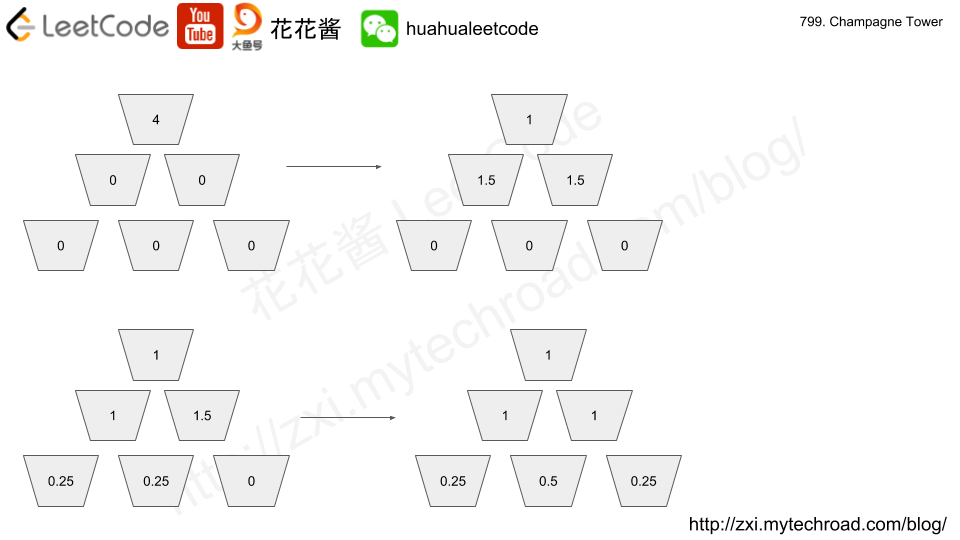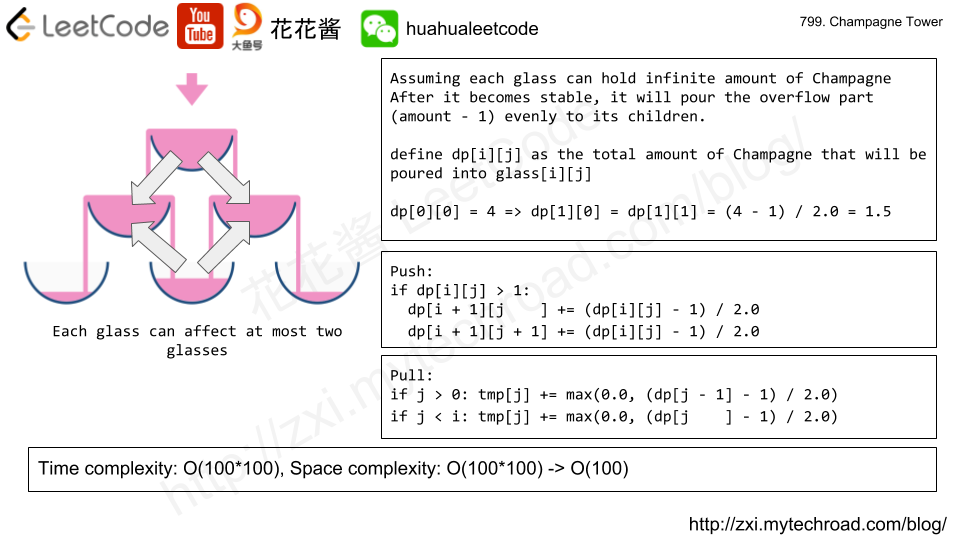Problem:

https://leetcode.com/problems/implement-stack-using-queues/description/

Implement the following operations of a stack using queues.

• push(x) — Push element x onto stack.
• pop() — Removes the element on top of the stack.
• top() — Get the top element.
• empty() — Return whether the stack is empty.

Notes:

• You must use only standard operations of a queue — which means only push to backpeek/pop from frontsize, and is empty operations are valid.
• Depending on your language, queue may not be supported natively. You may simulate a queue by using a list or deque (double-ended queue), as long as you use only standard operations of a queue.
• You may assume that all operations are valid (for example, no pop or top operations will be called on an empty stack).

Idea:

Using a single queue, for every push, shift the queue (n – 1) times such that the last element becomes the first element in the queue.

e.g.

push(1): q: 

push(2): q: [1, 2] -> [2, 1]

push(3): q: [2, 1, 3] -> [1, 3, 2] -> [3, 2, 1]

push(4): q: [3, 2, 1, 4] -> [2, 1, 4, 3] -> [1, 4, 3, 2] -> [4, 3, 2, 1]

Solution:

Time complexity:

Push: O(n)

Pop/top/empty: O(1)

Space complexity: O(n)

C++

Problem:

Given a singly linked list, determine if it is a palindrome.

Could you do it in O(n) time and O(1) space?

Idea:

1. use fast / slow pointers to find the middle node and see whether the list has odd/even number of elements.
2. Reverse the right half the list, and compare with the left half

E.g.
1->2->3->4->3->2->1->null
fast = null
slow = 4
slow->next = 3
reverse(slow->next)
null<-3<-2<-1 compare with 1->2->3->…

Solution: 1

Time complexity: O(n)

Space complexity: O(1)

C++

Problem:

https://leetcode.com/problems/champagne-tower/description/

We stack glasses in a pyramid, where the first row has 1 glass, the second row has 2 glasses, and so on until the 100th row.  Each glass holds one cup (250ml) of champagne.

Then, some champagne is poured in the first glass at the top.  When the top most glass is full, any excess liquid poured will fall equally to the glass immediately to the left and right of it.  When those glasses become full, any excess champagne will fall equally to the left and right of those glasses, and so on.  (A glass at the bottom row has it’s excess champagne fall on the floor.)

For example, after one cup of champagne is poured, the top most glass is full.  After two cups of champagne are poured, the two glasses on the second row are half full.  After three cups of champagne are poured, those two cups become full – there are 3 full glasses total now.  After four cups of champagne are poured, the third row has the middle glass half full, and the two outside glasses are a quarter full, as pictured below.Now after pouring some non-negative integer cups of champagne, return how full the j-th glass in the i-th row is (both i and j are 0 indexed.)

Note:

• poured will be in the range of [0, 10 ^ 9].
• query_glass and query_row will be in the range of [0, 99].Idea: DP + simulation

define dp[i][j] as the volume of champagne will be poured into the j -th glass in the i-th row, dp[i][j] can be greater than 1.

Init dp = poured.

Transition: if dp[i][j] > 1, it will overflow, the overflow part will be evenly distributed to dp[i+1][j], dp[i+1][j+1]

if dp[i][j] > 1:
dp[i+1][j] += (dp[i][j] – 1) / 2.0
dp[i+1][j + 1] += (dp[i][j] – 1) / 2.0

Solution 1:

C++

Time complexity: O(100*100)

Space complexity: O(100*100)

Pull

C++

Time complexity: O(rows * 100)

Space complexity: O(100)

Push

Pull

Problem:

https://leetcode.com/problems/all-paths-from-source-to-target/description

Given a directed, acyclic graph of N nodes.  Find all possible paths from node 0 to node N-1, and return them in any order.

The graph is given as follows:  the nodes are 0, 1, …, graph.length – 1.  graph[i] is a list of all nodes j for which the edge (i, j) exists.

Note:

• The number of nodes in the graph will be in the range [2, 15].
• You can print different paths in any order, but you should keep the order of nodes inside one path.

Solution 1: DFS

Time complexity: O(n!)

Space complexity: O(n)

“Cleaner” Version

Problem:

https://leetcode.com/problems/rotate-string/description/

We are given two strings, A and B.

shift on A consists of taking string A and moving the leftmost character to the rightmost position. For example, if A = 'abcde', then it will be 'bcdea' after one shift on A. Return True if and only if A can become B after some number of shifts on A.

Note:

• A and B will have length at most 100.

Solution 1: Brute Force

Time complexity: O(n^2)

Space complexity: O(1)

C++

Mission News Theme by Compete Themes.